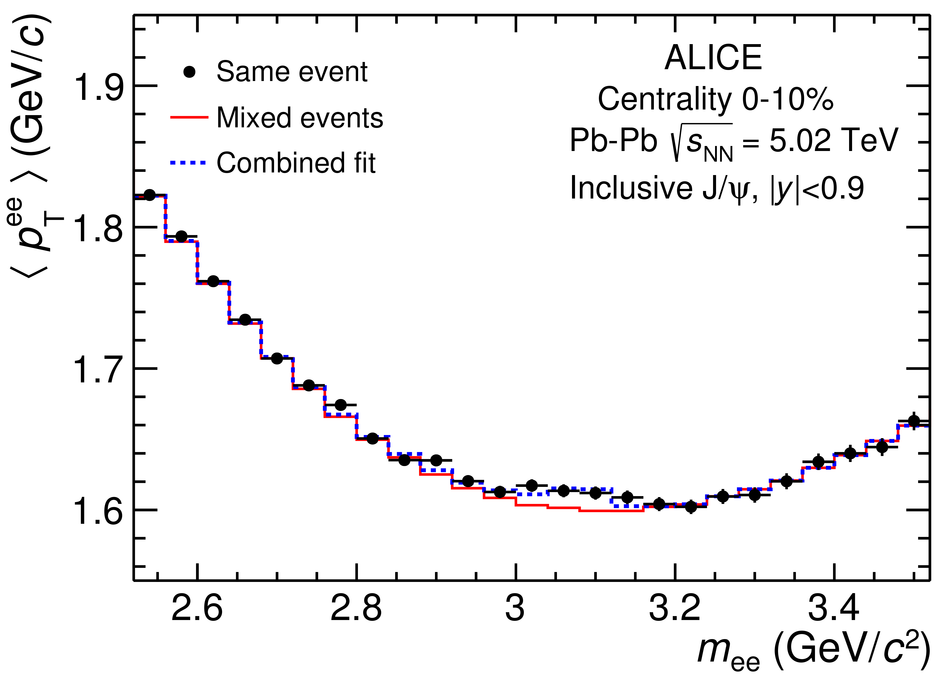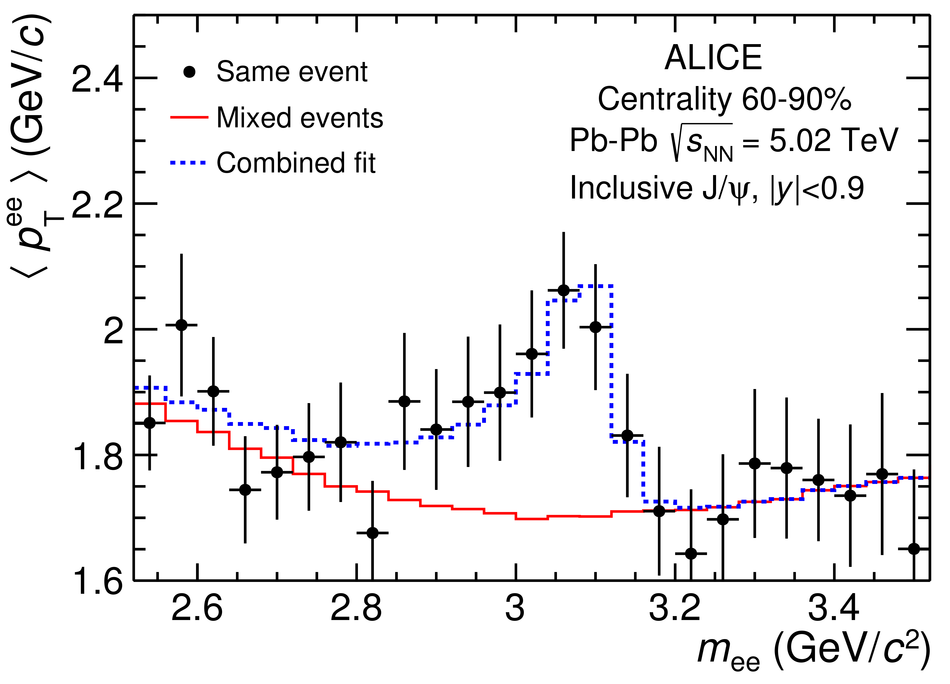# Figure 2

 Extraction of the $\jpsi$ $\MeanPt$ in $\PbPb$ collisions at $\snn = 5.02$ TeV for the $0$--$10$\% (left) and $60$--$90$\% (right) centrality classes in the transverse momentum interval $0.15< \pt< 10$\GeVc. The background, obtained from event-mixing, is shown by the red line.